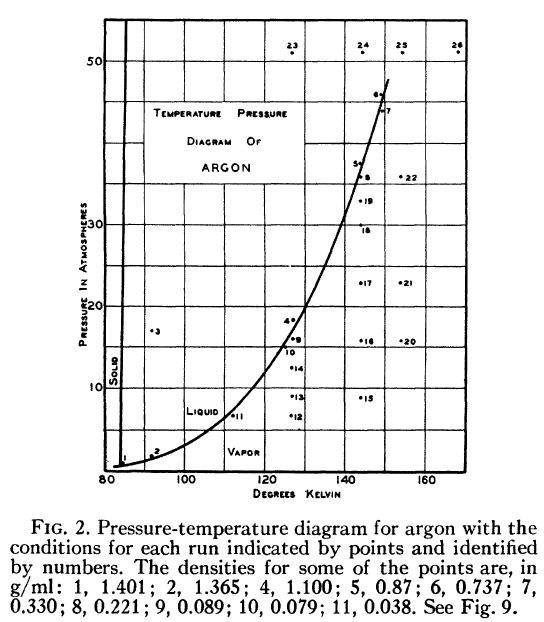# consultation on the pressure liquid argon lennard jones T=94.4K, rho=1.374 g/ml

Hello everyone ,

I simulating the liquid argon at 94.4K and a density of 1.374 g / ml . All is well so far, correctly I get the velocity distribution curves for the radial distribution function and diffusion coefficient(Rahman1964) .

roughly the code my script has the following structure:

1. Initial setup (rahman1964) boundary p p p
2. Geometry setup (rahman1964) lattice fcc
3. Run 100 steps:
{ run NVE and for every 10 time steps rescale speeds for temperature 94.4 K }
4. Unfix rescaling of velocities.
5. run 5000 steps : {
NVE only
}

However I have a question regarding the system pressure . plotting pressure vs. time . Meeting the pressure is - approx . 480 atom . However, looking at the diagram of pressure vs temperature of the article Eisenstein and company (1942 ) in argon at 94.4K and a density of 1,347g / ml in balance should obtain a pressure of about 10 atm approx.

Sending the pressure versus time graph obtained simulation and a screenshot of the article of the pressure diagram v / s temperature ( 1942).

Why my fluid pressure is so high compared to the experimental values ​​?
What can I be doing wrong ?

Regards

pressure.eps (28.5 KB)temperature.eps (28.4 KB)

Are you missing a long-range correction to the pressure, which will

otherwise vary with cutoff. See pair_modify tail for the option.

Steve